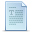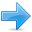# Namespaces

Namespaces allow to group entities like classes, objects and functions under a name. This way the global scope can be divided in "sub-scopes", each one with its own name.

The format of namespaces is:

namespace identifier
{
entities
}

Where identifier is any valid identifier and entities is the set of classes, objects and functions that are included within the namespace. For example:

 ``1234`` ``````namespace myNamespace { int a, b; }``````

In this case, the variables a and b are normal variables declared within a namespace called myNamespace. In order to access these variables from outside the myNamespace namespace we have to use the scope operator ::. For example, to access the previous variables from outside myNamespace we can write:

 ``12`` ``````myNamespace::a myNamespace::b``````

The functionality of namespaces is especially useful in the case that there is a possibility that a global object or function uses the same identifier as another one, causing redefinition errors. For example:

 ``````// namespaces #include using namespace std; namespace first { int var = 5; } namespace second { double var = 3.1416; } int main () { cout << first::var << endl; cout << second::var << endl; return 0; }`````` ```5 3.1416```

In this case, there are two global variables with the same name: var. One is defined within the namespace first and the other one in second. No redefinition errors happen thanks to namespaces.

### using

The keyword using is used to introduce a name from a namespace into the current declarative region. For example:

 ``````// using #include using namespace std; namespace first { int x = 5; int y = 10; } namespace second { double x = 3.1416; double y = 2.7183; } int main () { using first::x; using second::y; cout << x << endl; cout << y << endl; cout << first::y << endl; cout << second::x << endl; return 0; }`````` ```5 2.7183 10 3.1416```

Notice how in this code, x (without any name qualifier) refers to first::x whereas y refers to second::y, exactly as our using declarations have specified. We still have access to first::y and second::x using their fully qualified names.

The keyword using can also be used as a directive to introduce an entire namespace:

 ``````// using #include using namespace std; namespace first { int x = 5; int y = 10; } namespace second { double x = 3.1416; double y = 2.7183; } int main () { using namespace first; cout << x << endl; cout << y << endl; cout << second::x << endl; cout << second::y << endl; return 0; }`````` ```5 10 3.1416 2.7183```

In this case, since we have declared that we were using namespace first, all direct uses of x and y without name qualifiers were referring to their declarations in namespace first.

using and using namespace have validity only in the same block in which they are stated or in the entire code if they are used directly in the global scope. For example, if we had the intention to first use the objects of one namespace and then those of another one, we could do something like:

 ``````// using namespace example #include using namespace std; namespace first { int x = 5; } namespace second { double x = 3.1416; } int main () { { using namespace first; cout << x << endl; } { using namespace second; cout << x << endl; } return 0; }`````` ```5 3.1416```

### Namespace alias

We can declare alternate names for existing namespaces according to the following format:

namespace new_name = current_name;

### Namespace std

All the files in the C++ standard library declare all of its entities within the std namespace. That is why we have generally included the using namespace std; statement in all programs that used any entity defined in iostream.Previous:TemplatesIndexNext:Exceptions